# What Is the Sabathé Cycle? Description and Calculation of the Mixed Cycle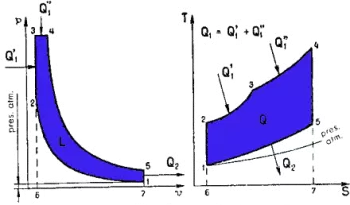The Sabathé cycle is a thermodynamic cycle for internal combustion engines in which combustion takes place partly at constant pressure and partly at constant volume. Sabathé cycle is also called Sabathé mixed cycle, dual combustion or limited or mixed pressure or Trinkler or Seiliger.

The actual operating conditions of diesel engines differ markedly from those represented in the ideal Otto and Diesel cycles. For diesel engines, the combustion process approaches a constant pressure transformation only in the case of exceptionally large and slow engines. Under normal conditions, the actual diagram shows that combustion takes place according to a process that approximates a transformation at constant volume and another at constant pressure.

It can be affirmed that, in practice, the Otto and Diesel cycles are very close in shape, to the point of being able to consider them as a particular case of the mixed cycle, in which part of the combustion takes place at constant volume, and part, at constant pressure.

## Description and Calculation of the Mixed Sabathé Cycle

In this cycle, after the adiabatic compression phase 1-2, a constant volume combustion phase 2-3 occurs. During this combustion phase the quantity of heat Q1 'is introduced and then, as in the Diesel cycle, a 3-4 phase of combustion at constant pressure, during which the quantity of heat Q1' 'is introduced.

Then two successive phases follow, namely: one, of adiabatic expansion 4-5, and another, of subtraction, at constant volume 5-1, of the quantity of heat Q2.

Therefore, the total amount of heat introduced is equal to:

Q 1 = Q 1 '+ Q 1 ' '

Recalling the above, regarding the Otto and Diesel cycles, we can write:

Q 1 '= C v  (T 3 -T 2 )

Q 1 '' = C p  (T 4 -T 3 )

Q 2 = C v  (T 5 -T 1 )

Thus, the ideal thermal performance of the theoretical Sabathé cycle is worth:

h e = (heat supplied - heat subtracted) / heat supplied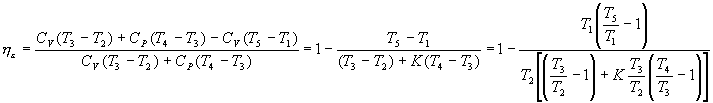For the 2-3 transformation of constant volume combustion we have: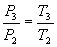And for the 3-4 transformation of constant pressure combustion: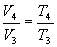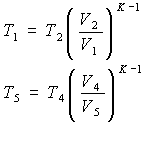For the adiabatic transformations 1-2 compression and 4-5 expansion we will use, respectively, the formulas

from which we obtain: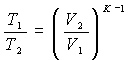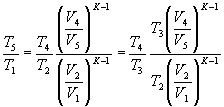and where V 3 = V 2   ; V 5 = V 1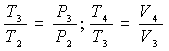It can be written: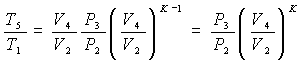Substituting these expressions for the ideal thermal performance, it results:Start with the relationship between the pressure P3 at the end and the pressure P2 at the beginning of the constant volume combustion phase - which we will call the “constant volume combustion ratio” -, and remembering that: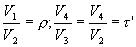the final expression of the  ideal thermal performance of the theoretical Sabathé cycle is obtained :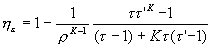## Sabathé Cycle Performance Conclusions

At the same compression ratio r, the performance of the mixed cycle is intermediate between that of the Otto cycle and that of the Diesel cycle. If the heat supplied at constant volume, that is, between points 2 and 3, is increased and the heat supplied at constant pressure between points 3 and 4 is reduced, the thermal efficiency approaches that of the Otto cycle. If, on the contrary, the heat supplied at constant volume is reduced and the corresponding one at constant pressure is increased, the performance of the mixed cycle approaches that of the Diesel cycle.

Author:

Published: March 31, 2011
Last review: February 18, 2020